Win up to 100% scholarship on Aakash BYJU'S JEE/NEET courses with ABNAT Win up to 100% scholarship on Aakash BYJU'S JEE/NEET courses with ABNAT

# Functions and Types of Functions

Functions are relations where each input has a particular output. This lesson covers the concepts of functions in Mathematics and the different types of functions using various examples for better understanding.

## JEE Main 2021 Maths LIVE Paper Solutions 24-Feb Shift-1 Memory-based## What Are Functions in Mathematics?

A function is a relation between a set of inputs and a set of permissible outputs with the property that each input is related to exactly one output. Let A & B be any two non-empty sets; mapping from A to B will be a function only when every element in set A has one end and only one image in set B.

Example: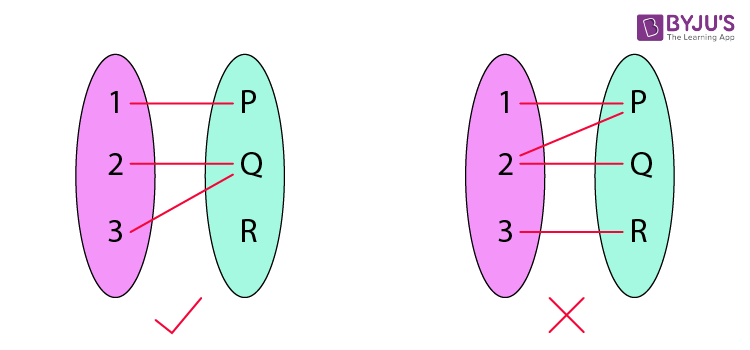Another definition of functions is that it is a relation “f” in which each element of set “A” is mapped with only one element belonging to set “B”. Also, in a function, there can’t be two pairs with the same first element.

### Condition for a Function

Set A and Set B should be non-empty.

In a function, a particular input is given to get a particular output. So, a function f: A->B denotes that f is a function from A to B, where A is a domain, and B is a co-domain.

• For an element, a, which belongs to A, a ∈ A, a unique element b, b ∈ B is there such that (a,b) f.

The unique element b to which f relates a is denoted by f(a) and is called f of a, or the value of f at a, or the image of a under f.

• The range of (image of a under f)
• It is the set of all values of f(x) taken together.
• Range of f = { y Y | y = f (x), for some x in X}

A real-valued function has either P or any one of its subsets as its range. Further, if its domain is also either P or a subset of P, it is called a real function.

Vertical Line Test:

A vertical line test is used to determine whether a curve is a function or not. If any curve cuts a vertical line at more than one point, then the curve is not a function.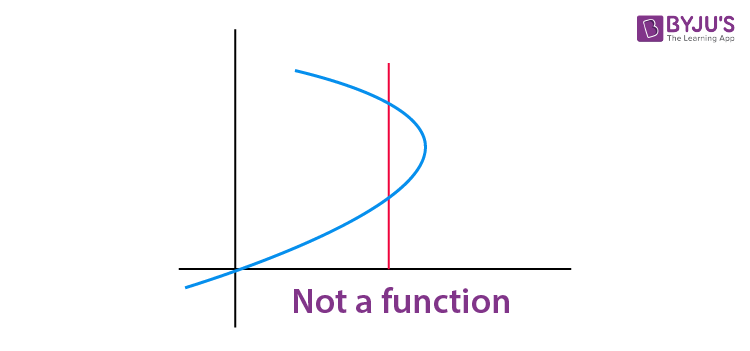### Representation of Functions

Functions are generally represented as f(x).

Let , f(x) = x3.

It is said as f of x is equal to x cube.

Functions can also be represented by g(), t(),… etc.

### Steps for Solving Functions

Question: Find the output of the function g(t) = 6t2 + 5 at

(i) t = 0

(ii) t = 2

Solution:

The given function is g(t) = 6t2 + 5

(i) At t = 0, g(0) = 6(0)2 + 5 = 5

(ii) At t = 2, g(2) = 6(2)2 + 5 = 29

## Types of Functions

There are various types of functions in Mathematics which are explained below in detail. The different function types covered here are given below:

• One-one Function (Injective Function)
• Many-one Function
• Onto Function (Surjective Function)
• Into Function
• Polynomial Function
• Linear Function
• Identical Function
• Rational Function
• Algebraic Functions
• Cubic Function
• Modulus Function
• Signum Function
• Greatest Integer Function
• Fractional Part Function
• Even and Odd Function
• Periodic Function
• Composite Function
• Constant Function
• Identity Function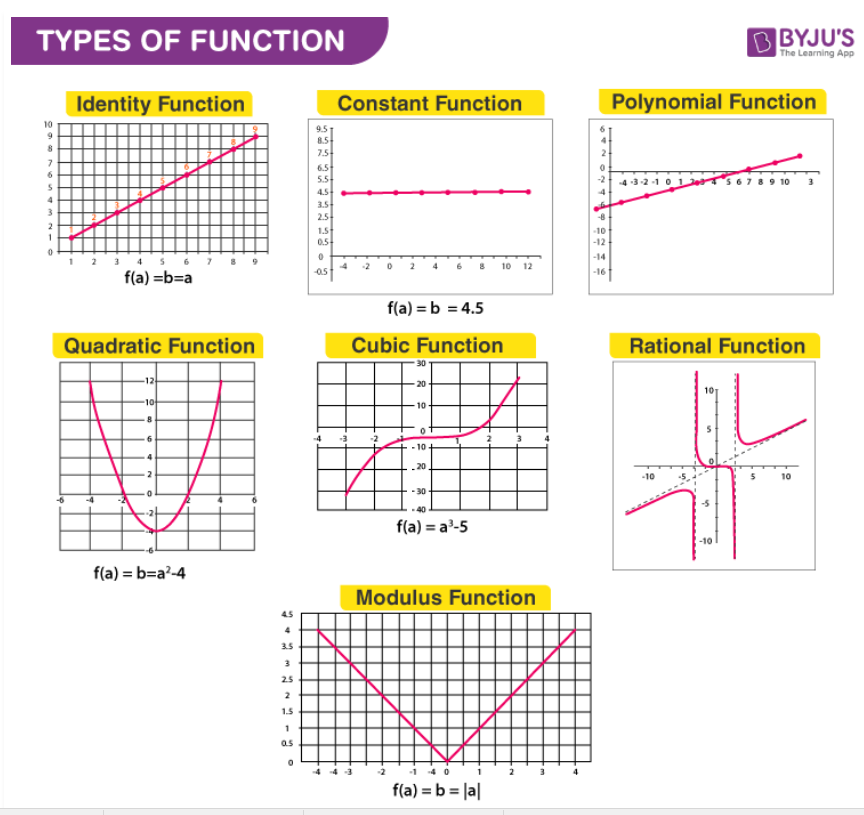Practice: Find the missing equations from the above graphs.

## Functions and Types of Functions## Number of Functions## Even and Odd Functions## Composite and Periodic Functions### One-one Function (Injective Function)

If each element in the domain of a function has a distinct image in the co-domain, the function is said to be a one-one function.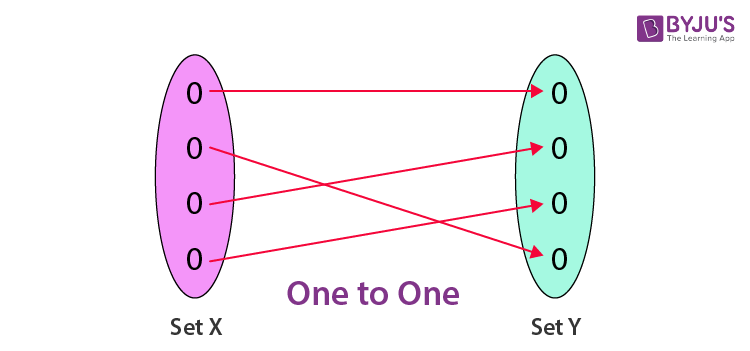For example, f; R R, given by f(x) = 3x + 5, is one-one.

### Many-one function

On the other hand, if there are at least two elements in the domain whose images are the same, the function is known as many to one.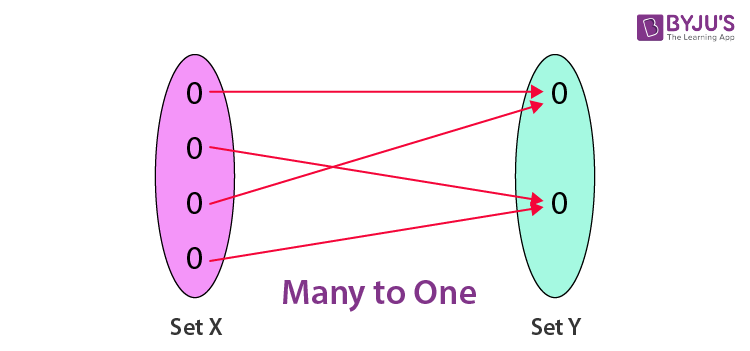For example, f : R R, given by f(x) = x2 + 1, is many-one.

### Onto Function (Surjective Function)

A function is called an onto function if each element in the co-domain has at least one pre-image in the domain.

### Into Function

If there exists at least one element in the co-domain, which is not an image of any element in the domain, then the function will be into function.

(Q) Let A = {x : 1 < x < 1} = B be a mapping f : A B; find the nature of the given function (P). F(x) = |x|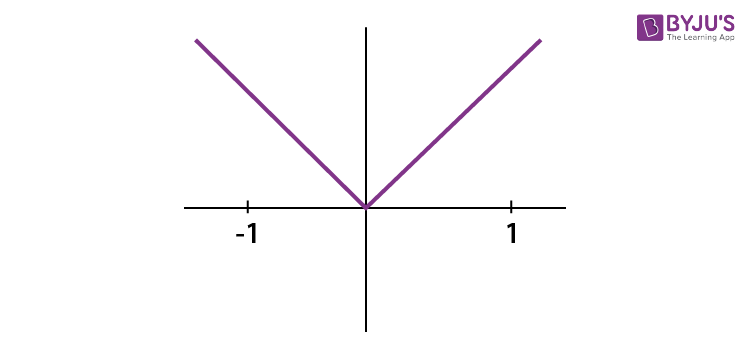f (x) = |1|

Solution for x = 1 & -1

Hence, it is many one, the range of f(x) from [-1, 1] is [0, 1] is not equal to the co-domain.

Hence, it is into function.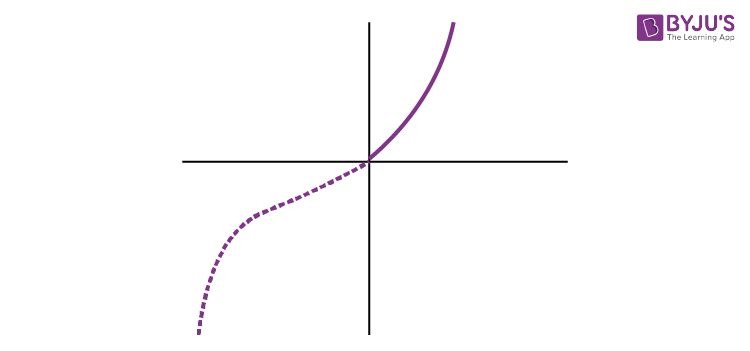Let’s say we have the function,

$$\begin{array}{l}f(x)=\left\{\begin{matrix} x^2 & ; & x\geq 0\\ -x^2 & ; & x<0 \end{matrix}\right.\end{array}$$

For different values of the input, we have different outputs; hence it is a one-one function, and also, it manages equally to its co-domain; hence, it is onto also.

### Polynomial function

A real-valued function f : P → P defined by

$$\begin{array}{l}y = f(a) = h_{0}+h_{1}a+…..+h_{n}a^{n}\end{array}$$
, where n ∈ N and h0 + h1 + … + hn ∈ P, for each a ∈ P, is called polynomial function.

• N = a non-negative integer.
• The degree of the polynomial function is the highest power in the expression.
• If the degree is zero, it’s called a constant function.
• If the degree is one, it’s called a linear function. For example, b = a+1.
• Graph type: Always a straight line.

So, a polynomial function can be expressed as:

$$\begin{array}{l}f(x)= a_{n}x^{n}+a_{n-1}x^{n-1}+…..+a_{1}x^{1}+a_{0}\end{array}$$

The highest power in the expression is known as the degree of the polynomial function. The different types of polynomial functions based on the degree are as follows:

1. The polynomial function is called a constant function if the degree is zero.
2. The polynomial function is called a linear if the degree is one.
3. The polynomial function is quadratic if the degree is two.
4. The polynomial function is cubic if the degree is three.

### Linear Function

All functions in the form of ax + b where a, b ∈ R & a ≠ 0 are called linear functions. The graph will be a straight line. In other words, a linear polynomial function is a first-degree polynomial where the input needs to be multiplied by m and added to c. It can be expressed by f(x) = mx + c.

For example, f(x) = 2x + 1 at x = 1

f(1) = 2.1 + 1 = 3

f(1) = 3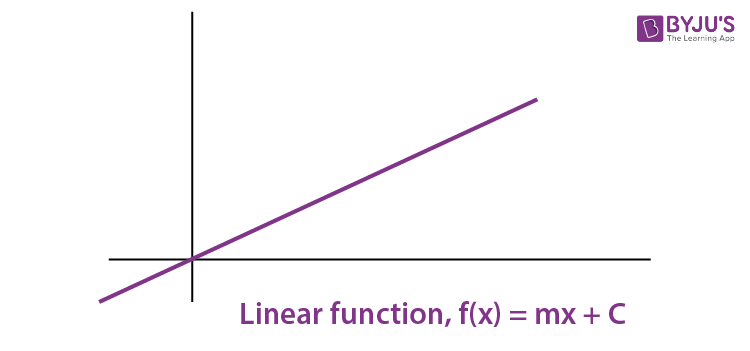Another example of a linear function is y = x + 3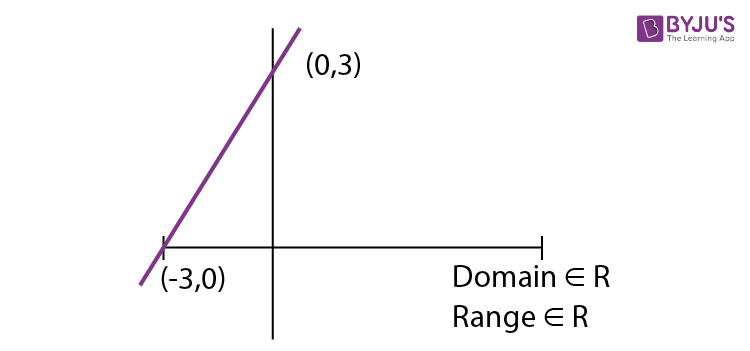### Identical Function

Two functions, f and g, are said to be identical if

(a) The domain of f = domain of g

(b) The range of f = the range of g

(c) f(x) = g(x) ∀ x ∈ Df & Dg

For example, f(x) = x

$$\begin{array}{l}g(x) = \frac{1}{1/x}\end{array}$$

Solution: f(x) = x is defined for all x

But

$$\begin{array}{l}g(x) = \frac{1}{1/x}\end{array}$$
is not defined of x = 0

Hence, it is identical for x ∈ R – {0}

All functions in the form of y = ax2 + bx + c where a, b, c ∈ R, a ≠ 0 will be known as Quadratic functions. The graph will be parabolic.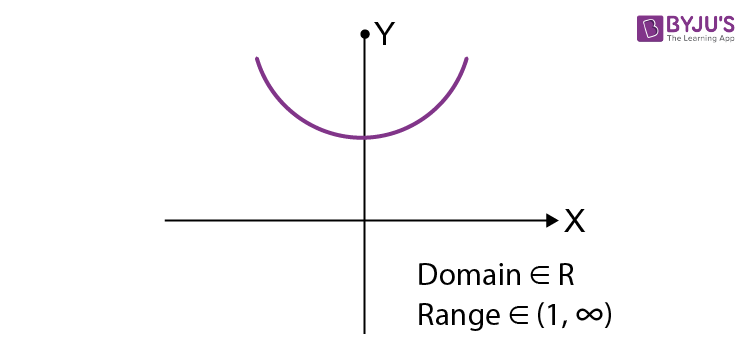$$\begin{array}{l}\text{At}\ x=\frac{-b \pm \sqrt{D}}{2}\end{array}$$
, we will get its maximum on minimum value depending on the leading coefficient, and that value will be -D/4a (where D = Discriminant).

In simpler terms,

A quadratic polynomial function is a second-degree polynomial, and it can be expressed as:

F(x) = ax2 + bx + c, and a is not equal to zero.

Where a, b, and c are constant, and x is a variable.

Example, f(x) = 2x2 + x – 1 at x = 2

If x = 2, f(2) = 2.22 + 2 – 1 = 9

For example, y = x2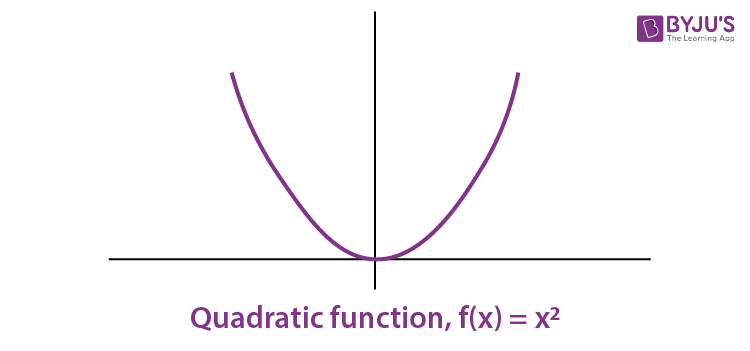### Rational Function

These are the real functions of the type

$$\begin{array}{l}\frac{f(a)}{g(a)}\end{array}$$
where f (a) and g (a) are polynomial functions of a defined in a domain, where g(a) ≠ 0.

• For example, f : P – {– 6} → P defined by
$$\begin{array}{l}f(a) = \frac{f(a+1)}{g(a+2)}\forall a\in P – {-6},\end{array}$$
is a rational function.
• Graph type: Asymptotes (the curves touching the axes lines).

### Algebraic Functions

An algebraic equation is known as a function that consists of a finite number of terms involving powers and roots of independent variable x and fundamental operations, such as addition, subtraction, multiplication and division.

For example,

$$\begin{array}{l}f(x)=5x^{3}-2x^{2}+3x+6\end{array}$$
,
$$\begin{array}{l}g(x)=\frac{\sqrt{3x+4}}{(x-1)^{2}}\end{array}$$
.

### Cubic Function

A cubic polynomial function is a polynomial of degree three and can be expressed as:

F(x) = ax3 + bx2 + cx + d, and a is not equal to zero.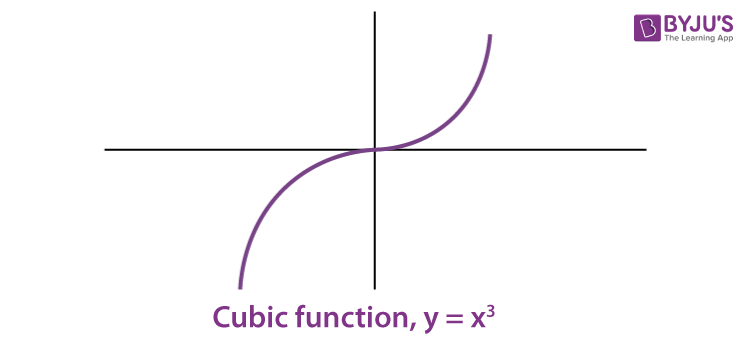In other words, any function in the form of f(x) = ax3 + bx2 + cx + d, where a, b, c, d ∈ R & a ≠ 0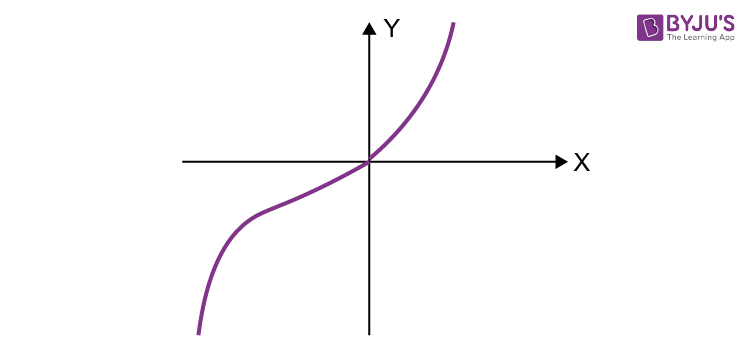For example, y = x3

Domain R

Range R

### Modulus Function

The real function f : P → P defined by f (a) = |a| = a when a ≥ 0. and f(a) = -a when a < 0 ∀ a ∈ P is called the modulus function.

• Domain of f = P
• Range of f = P+ U {0}
$$\begin{array}{l}y=|x|=\left\{\begin{matrix} x & x\geq 0\\ -x & x<0 \end{matrix}\right.\end{array}$$

Domain: R

Range: [0, ∞)

### Signum Function

The real function f : P → P is defined by

$$\begin{array}{l}\left\{\begin{matrix}\frac{\left | f(a) \right |}{f(a)}, a\neq 0 \\ 0, a=0 \end{matrix}\right. = \left\{\begin{matrix} 1,if x>0\\ 0, if x=0\\ -1, if x<0\end{matrix}\right.\end{array}$$

is called the signum function or sign function (gives the sign of real number).

• Domain of f = P
• Range of f = {1, 0, – 1}

For example, signum (100) = 1

signum (log 1) = 0

signum (x21) =1

### Greatest Integer Function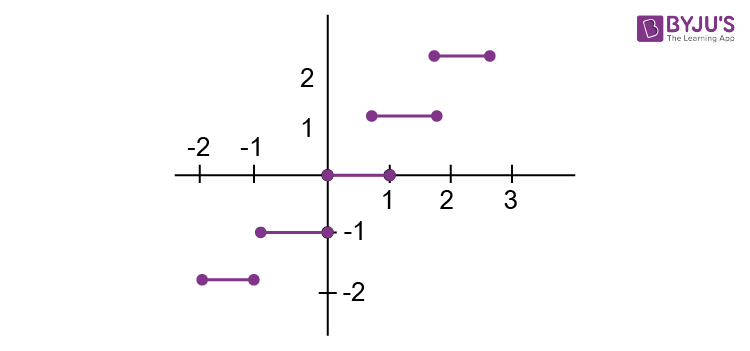The real function f : P → P defined by f (a) = [a], a  P assumes the value of the greatest integer less than or equal to a is called the greatest integer function.

• Thus f (a) = [a] = – 1 for – 1 ⩽ a < 0
• f (a) = [a] = 0 for 0 ⩽ a < 1
• [a] = 1 for 1 ⩽ a < 2
• [a] = 2 for 2 ⩽ a < 3 and so on…

The greatest integer function always gives integral output. The greatest integral value that has been taken by the input will be the output.

For example, [4.5] = 4

[6.99] = 6

[1.2] = 2

Domain R

Range Integers

### Fractional Part Function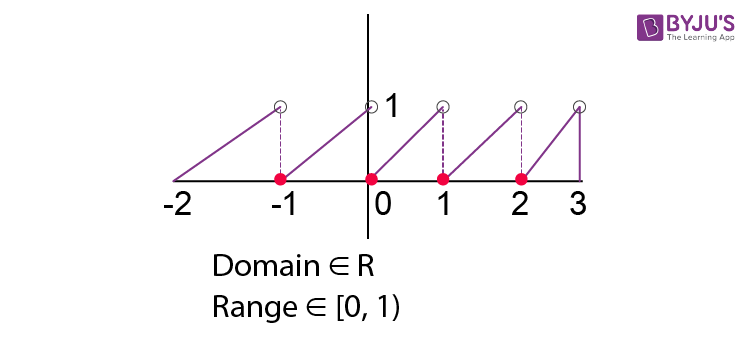{x} = x – [x]

It always gives fractional value as output.

For example, {4.5} = 4.5 – [4.5]

= 4.5 – 4 = 0.5

{6.99} = 6.99 – [6.99]

= 6.99 – 6 = 0.99

{7} = 7 –  = 7 –7 = 0

### Even and Odd Function

If f(x) = f(-x), then the function will be even function, and if f(x) = -f(-x), then the function will be odd function.

Example 1:

f(x) = x2sinx

f(-x) = -x2sinx

Here, f(x) = -f(-x)

It is an odd function.

Example 2:

$$\begin{array}{l}f(x)={{x}^{2}}\end{array}$$

and

$$\begin{array}{l}f(-x)={{x}^{2}}\end{array}$$

f(x) = f(-x)

It is an even function.

### Periodic Function

A function is said to be a periodic function if a positive real number T exists, such that f(u – t) = f(x) for all x ε domain.

For example, f(x) = sin x

f(x + 2π) = sin (x + 2π) = sin x fundamental

Then period of sin x is 2π.

### Composite Function

Let A, B, and C be three non-empty sets

Let f: A B & g : G C be two functions, then gof : A C. This function is called the composition of f and g given gof (x) = g(f(x)).

For example, f(x) = x2 & g(x) = 2x

f(g(x)) = f(2,x) = (2x)2 = 4x2

g(f(x)) = g(x2) = 2x2

### Constant Function

The function f : P → P is defined by b = f (x) = D, a P, where D is a constant P, is a constant function.

• Domain of f = P
• Range of f = {D}
• Graph type: A straight line which is parallel to the x-axis.

In simple words, the polynomial of 0th degree where f(x) = f(0) = a0 = c. Regardless of the input, the output always results in a constant value. The graph for this is a horizontal line.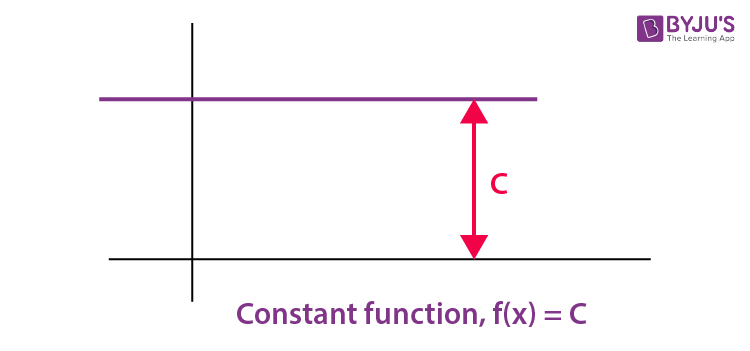### Identity Function

P= set of real numbers

The function f : P → P defined by b = f (a) = a for each a P is called the identity function.

• Domain of f = P
• Range of f = P
• Graph type: A straight line passing through the origin.

## Functions Video

### Domain, Range, Period of Functions### Functions and Relations## Relations and Functions Questions## One-One and Onto FunctionsQ1

### What do you mean by a function in Mathematics?

A relation f from set A to set B is called a function if every element of set A has one and only one image in set B.

Q2

### What do you mean by domain of a function?

The domain of a function is the set of all possible inputs for a function.

Q3

### What do you mean by range of a function?

The range of a function is the set of all possible output values.

Q4

### What do you mean by a constant function?

Constant function is a function whose output is the same for every input value. For example, f(x) = 3. Here, for every value of x, output will be 3.

Test your knowledge on Functions And Its Types